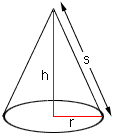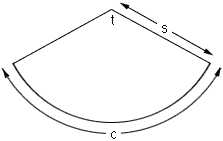Subject: paper cone Date: Sat, 29 Jul 2000 14:33:29 -0400 Hi I am a grand parent 75 years old. The question of how to lay out & cut out of paper, cones came up. I would like the cone have : A base of 4 inches and height of 4 inches, 6 inches, 8 inches. Thank you, John Hi John, Suppose that you have a cone of height h, slant height s, and the radius of the base r.Figure 1 From the right triangle in Figure 1, s2 = h2 + r2. Since you asked for "A base of 4 inches" I am going to assume that r = 2. Thus s is the square root of h2 +22. If you slice up the side of the cone and roll it out flat you can see the shape that is required to make the cone. It is a sector of a circle of radius s, the slant height of the cone, and arclength c, the circumference of the circular base of the cone. That is c = 2 pi r.Figure 2 From Figure 2, c is an arc of a circle of radius s determined by an angle of to so c = (pi s t)/180, and thus t = (180 c)/(pi s) degrees. Hence, calculate s for each of the four values of the height h using s is the square root of h2 +22. Draw a circle of radius s, cut out a sector with angle (180 c)/(pi s) degrees and roll it up to form the required cone. Cheers, Harley Go to Math Central